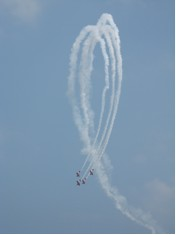# Mechanics: Circular Motion and Gravitation

## Circular Motion and Gravitation: Audio Guided Solution

#### Problem 10:A 76-kg pilot at an air show performs a loop de loop with his plane. At the bottom of the 52-m radius loop, the plane is moving at 48 m/s. Determine the normal force acting upon the pilot.

## Habits of an Effective Problem Solver

• Read the problem carefully and develop a mental picture of the physical situation. If necessary, sketch a simple diagram of the physical situation to help you visualize it.
• Identify the known and unknown quantities in an organized manner. Equate given values to the symbols used to represent the corresponding quantity - e.g., m = 61.7 kg, v= 18.5 m/s, R = 30.9 m, Fnorm = ???.
• Use physics formulas and conceptual reasoning to plot a strategy for solving for the unknown quantity.
• Identify the appropriate formula(s) to use.
• Perform substitutions and algebraic manipulations in order to solve for the unknown quantity.